Function Repository Resource:

# ExtendedApart

A partial fraction decomposition with the Extension option

Contributed by: Wolfram Staff (part of original concept by Dan McDonald)
 ResourceFunction["ExtendedApart"][exp,var] rewrites a rational expression as a sum of terms with minimal degree denominators in the complex domain, treating all variables other than var as constants. ResourceFunction["ExtendedApart"][exp,var,Extension→{a1,a2,…}] allows coefficients that are rational combinations of the algebraic numbers ai.

## Details and Options

Similar to Apart, ResourceFunction["ExtendedApart"] gives the partial fraction decomposition of a rational expression.
ResourceFunction["ExtendedApart"] essentially adds the standard Extension option to Apart, with the default value .
The possible values of the Extension option are the same as in Factor.

## Examples

### Basic Examples (2)

Decompose a rational expression into partial fractions:

 In:=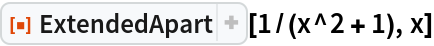Out=Compare with Apart:

 In:=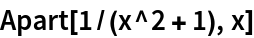Out=### Scope (5)

Factor the denominator into linear factors:

 In:=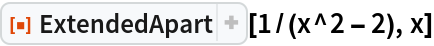Out=Decompose allowing complex coefficients:

 In:=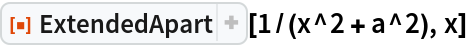Out=In:=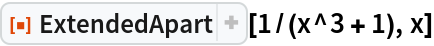Out=In:=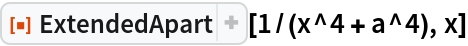Out=In:=Out=Allow the rationals extended by the algebraic number coefficients:

 In:=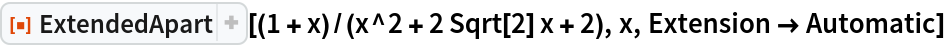Out=Allow a finite algebraic extension of rationals:

 In:=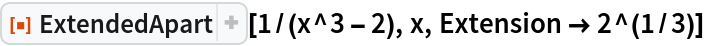Out=In:=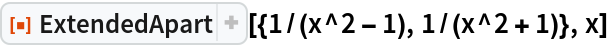Out=### Properties and Relations (2)

For some expressions, ExtendedApart gives results similar to Apart[Factor[exp]], with a proper Extension option for Factor:

 In:=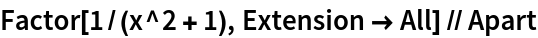Out=In:=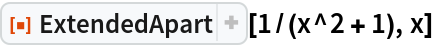Out=does not necessary uses the smallest denominators:

 In:=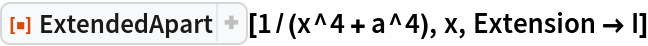Out=Compare:

 In:=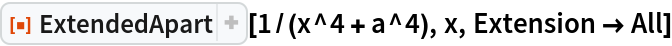Out=## Version History

• 1.0.0 – 20 November 2020